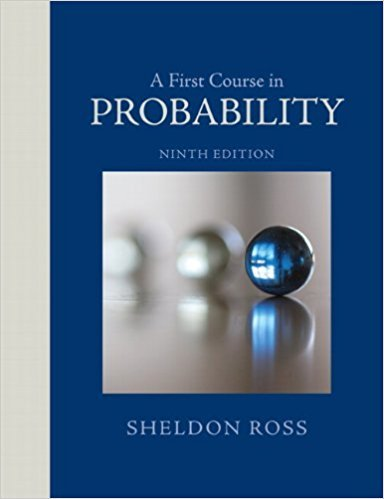×
Get Full Access to A First Course In Probability - 9 Edition - Chapter 3 - Problem 77p
Get Full Access to A First Course In Probability - 9 Edition - Chapter 3 - Problem 77p

×

# Consider an unending sequence of independent trials, whereISBN: 9780321794772 63

## Solution for problem 77P Chapter 3

A First Course in Probability | 9th Edition

• Textbook Solutions
• 2901 Step-by-step solutions solved by professors and subject experts
• Get 24/7 help from StudySoup virtual teaching assistantsA First Course in Probability | 9th Edition

4 5 1 332 Reviews
16
3
Problem 77P

Problem 77P

Consider an unending sequence of independent trials, where each trial is equally likely to result in any of the outcomes 1, 2, or 3. Given that outcome 3 is the last of the three outcomes to occur, find the conditional probability that

(a) the first trial results in outcome 1;

(b) the first two trials both result in outcome 1.

Step-by-Step Solution:

Solution:

Step 1 of 3:

It is given that each trial is equally likely to result any one of the outcomes 1,2,3 in a unending sequence of independent trials.

Also, it is given that 3 is last outcome to occur.

Using this we need to find the required conditional probabilities.

Step 2 of 3

Step 3 of 3

##### ISBN: 9780321794772

Unlock Textbook Solution